Initial Forces Created Edited

# Initial Forces Control Data

## Function

• Initial forces, which are defined in Load > Initial Forces > Small Displacement > Initial Element Forces, are added to a specific load case. Or initial member forces are generated from Member forces due to a specific load combination.
• For the backward stage analysis of a cable stayed bridge, the nodal forces of the completed structure subjected to tension and dead loads are calculated. This makes possible to consider the zero displacement condition while the internal forces exist in the structure.
• Also, by considering member forces induced by the self weight of a structure during a pushover analysis, we can avoid creating another time history load case for the self weight.
• The calculated nodal forces and member forces can be checked in tabular form in "Equilibrium Element Nodal Force" and "Initial Element Force."
• The nodal forces are found after multiplying the nodal displacement and the stiffness of the structure, and the member forces are obtained by adding the fixed end forces (moments) to the nodal forces.
• "Initial Force Combination" and "Initial Element Forces" cannot be used simultaneously. "Initial Force Combination" calculates the initial member forces generated by the selected load. In "Initial Element Forces," you can specify whether to incorporate the calculated initial member forces from "Initial Force Combination" into the member forces of the selected load case.

## Call

From main menu, select [Load] tab > [Type : Static Loads] > [Initial Forces/Etc.] group > [Initial Forces] > [Small Displacement] >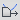[Initial Forces Control Data]

## Input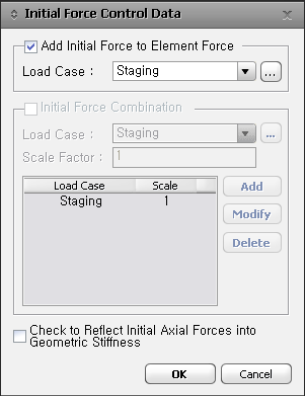Initial Force Control Data dialog box

### Add Initial Force to Element Force

The initial forces are reflected in the member forces and nodal reactions of the load case for which the user specifies the initial forces.

Load Case : Select the load case that reflects the Initial Force.

### Initial Force Combination

Load Case : Select and enter load cases for which initial axial forces are to be saved or added.

Scale Factor : Enter a scale factor for each load case.

Enter the relevant load case and scale factor, and click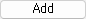.

Case 2 : To modify previously entered load case

After selecting the relevant load case, modify the scale factor, and click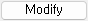.

Case 3 : To delete previously entered load case

Select the relevant load case, and click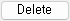.

### Check to Reflect Initial Axial Forces into Geometric Stiffness

Check if Initial Axial Forces are considered.

0